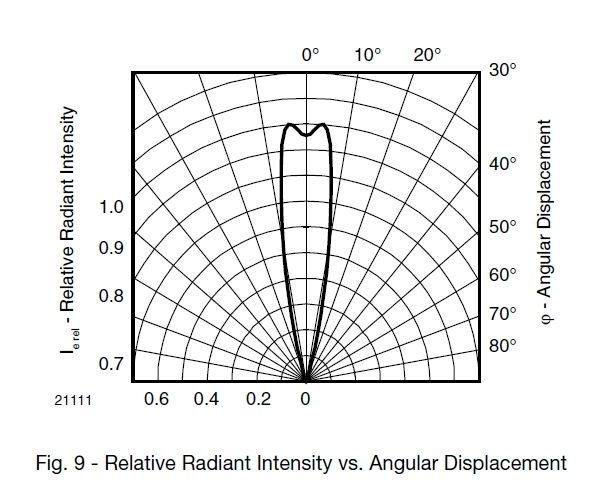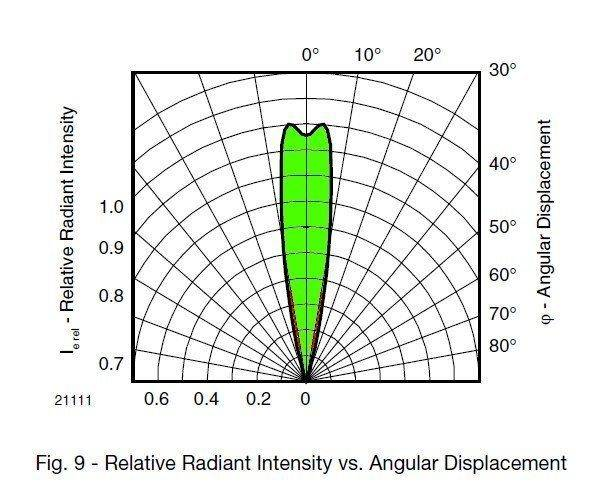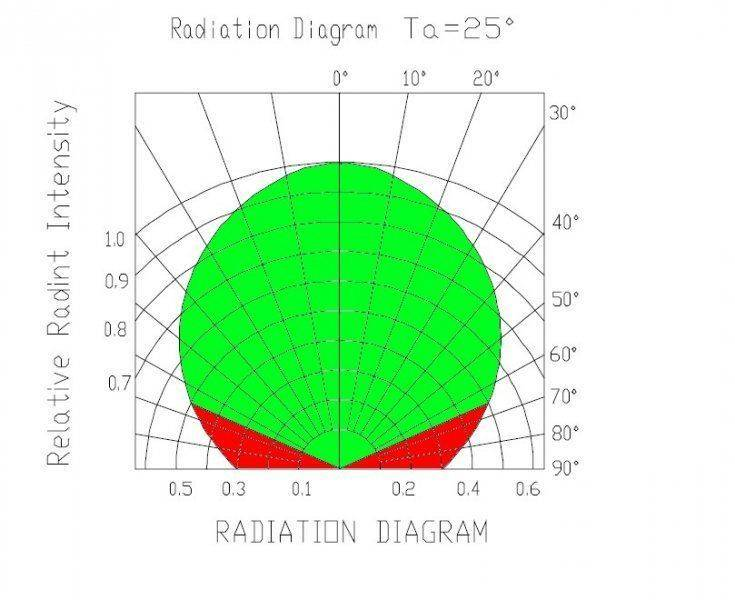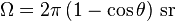Hi all,

I have a problem of understanding the concept of radiant intensity,
in particularly I’m interested in LED characteristics and confused with specification in LED datasheet.
For example in the datasheeat for the VSMY2850 LED from Vishay it is stated that typical
radiant intensity is 100 mW/sr. So if I multiply this number by the full solid angle 4pi
I should get the total power radiated by that LED, right ?
But this is obviously not the case, since the stated radiant power is 55mW.

Drakkith
Staff Emeritus
Is 4pi correct? I don't think the LED lights up a sphere, but a cone.

Andy Resnick
<snip>
For example in the datasheeat for the VSMY2850 LED from Vishay it is stated that typical
radiant intensity is 100 mW/sr. <snip>.

According to the datasheet: "Angle of half intensity: φ = ± 10°", corresponding to 0.38 sr. For more specific calculations, you need a better sense of how the intensity is distributed in angle, but 55 mW total radiated power seems reasonable.

So is it safe to assume that almost all of this this 55mW is radiated in an Angle of half intensity then ?

Andy Resnick
Definitely not- the angle of half intensity is conceptually similar to a 'full width- half maximum' specification. Light is emitted outside of the ± 10° cone angle.

CWatters
Homework Helper
Gold Member
Andy is right. It's not safe to assume that almost all of the power is emitted in the angle of half intensity. However if you look at the plot for the VSMY2850 it's perhaps not unreasonable in this case. Depends how accurate you need to be.Andy is right. It's not safe to assume that almost all of the power is emitted in the angle of half intensity. However if you look at the plot for the VSMY2850 it's perhaps not unreasonable in this case. Depends how accurate you need to be.
View attachment 78700

Ok, so this LED has 55mW Total radiant power (typical).
From the plot I can see that for relative intensity values > 0.1 the whole plot is within 20° half angle,
can I now assume that 90% of emitted 55mW is within 20° half angle ?
Is it then correct to say that within 10° half angle the emitted power will be 50% of the 55mW ?

CWatters
Homework Helper
Gold Member
It's a long time since I did this but if my memory is correct it's the volume under the curve that matters. Volume not area because the curve is actually in 3D. For example to calculate the power within the 10 degree half intensity you have to compare the green volume with the red volume. So in this case it looks like a very high percentage is emitted within the half intensity angle. Safe to assume near 100% I'd say (especially if you take into account the tolerance of the power output?)If someone knows better let me know!

CWatters
Homework Helper
Gold Member
For this LED it might not be safe to ignore the power outside the half intensity angle...Andy Resnick
Ok, so this LED has 55mW Total radiant power (typical).
From the plot I can see that for relative intensity values > 0.1 the whole plot is within 20° half angle,
can I now assume that 90% of emitted 55mW is within 20° half angle ?
Is it then correct to say that within 10° half angle the emitted power will be 50% of the 55mW ?

Let's get back to 'radiant intensity'- given what's been discussed already, do you understand some basic differences between say, irradiance (mW/cm^2) and intensity (mW/sr)?

Let's get back to 'radiant intensity'- given what's been discussed already, do you understand some basic differences between say, irradiance (mW/cm^2) and intensity (mW/sr)?

In the case of the above LED (suppose radiation pattern rotationally symmetric in 3D), if I have a surface
which subtends a conical solid angle of ±10° on the optical axis, the irradiance would drop
as the surface is moved away from the source. Radiant intensity would not change,
moreover, I can count on the total power of 55mW in that solid angle.

For this LED it might not be safe to ignore the power outside the half intensity angle...
View attachment 78730

CWatters, thanks a lot for the pictures, I think I can now understand the relationship between the half intensity angle, the total power and how to read out where I can find that power from the radiation pattern plots of the LED's.
Now, I just need to connect this with Radiant intensity.

I still do not understand how to get 100 mW/sr of typical radiant intensity from typical power of 55 mW for the VSMY2850 above. ±10° half angle gives me 0.1 sr

Ω = A/r2 = (Sin210*r2*π)/r2 = 0.1

Since for this LED all power is within the half angle, the radiant intensity should be 55 mW / 0.1 sr = 550 mW/sr.

Andy, how did you calculate 0.38 sr for the solid angle ?
55 mW / 0.38 sr = 145 mW/sr, looks better (so I guess my solid angle is wrong). But this is still closer to maximum rating then typical.

Andy Resnick
In the case of the above LED (suppose radiation pattern rotationally symmetric in 3D), if I have a surface which subtends a conical solid angle of ±10° on the optical axis, the irradiance would drop as the surface is moved away from the source. Radiant intensity would not change, moreover, I can count on the total power of 55mW in that solid angle.

Yes, ok. And the reason the irradiance decreases is because the surface much becomes larger in order to subtend a constant solid angle.

Andy, how did you calculate 0.38 sr for the solid angle ?
55 mW / 0.38 sr = 145 mW/sr, looks better (so I guess my solid angle is wrong). But this is still closer to maximum rating then typical.

Good catch- I used the wrong angle; the formula for the solid angle of a cone whose cross-section subtends the angle 2θ is:.

which results in 0.1 sr. I guess I don't understand what your goal is; you have a spec for the total radiated power and some qualitative information about the angular distribution of intensity- for example, you don't have the peak intensity (at θ = 0), nor do you have the function I(θ) (technically speaking, you don't have I(θ,φ), but I think you can assume the beam is axisymmetric). Are you simply trying to show that the spec is internally consistent?

- what you can do is essentially solve ∫I(θ,φ) dΩ = 2π∫I(θ)d(cosθ) using your boundary conditions: I(0) = 100 mW/sr and 55mW = 2π∫I(θ)d(cosθ) where the integral is over the full 2π hemisphere to get a function I(θ), If that's what you are thinking of doing...

Last edited:
CWatters
Homework Helper
Gold Member
I think I see his point. The data sheet gives the "Radiant Power as 55mW". The plot of intensity vs angle suggests the vast majority of the power is emitted within the 10 degree half intensity angle (=0.1sr). If you assume that the 55mW is distributed uniformly wouldn't that mean the Radiant Intensity is at least ...

55mW/0.1sr = 550mW/sr ??

the data sheet says the Radiant Intensity is only 100mW/sr.

If the beam is non-uniform I can see how that might make some parts higher but not lower.

This suggests we are converting from Radiant Power to Intensity incorrectly or the data sheet is wrong?

How would it work if the beam was uniformly distributed over the 10 degree angle?

Andy Resnick
<snip>How would it work if the beam was uniformly distributed over the 10 degree angle?

Going from the graph in Post #6, the intensity is (essentially) at it's maximal value within ±4° (0.015 sr), so that central portion of illumination only carries 1.5 mW.

Now the spec sheet seems a little odd- they quote a maximum radiated power of 55 mW, but they give various values for the radiant intensity (50 mW/sr, 100 mW/sr, 150 mW/sr) for 100 mA drive current, but they don't say if that's the value for θ=0 or not. Let's assume that's what they mean- the maximum intensity is typically 100 mW head-on (which is how I got 1.5 mW above).

But here's where I ran into a problem: from the I(θ) chart, I= 0 around θ=0.35 rad, so 2π∫I(θ)d(cosθ) = 55 mW, or if I write I(θ) = 100 mW/sr *f(θ), then f(0) = 1 and f(0.35) = 0. The intensity integral ∫f(θ)d(cosθ) = 0.0875 and I can't get it to match up for any simple function f(θ). It's possible I'm using the wrong angle θ, since sin(0)=0 and that's where the maximum intensity is- maybe the correct limits are π/2 - 0.35 < θ < π/2 instead of 0< θ < 0.35.

At this point, I would recommend contacting the company and simply ask for I(θ) rather than running around in circles.

 I couldn't stop obsessing. Yes, once I oriented the angle θ correctly, everything worked out. Given a total power of 55 mW and a f(θ)∝sin2(θ), I got a peak intensity of 180 mW/sr, and the half intensity correctly located at θ=0.17 rad, with a emitted intensity within that cone of 14.8 mW. So either their total radiated power spec is a little off (for a peak I = 150 mW/sr, the total radiated power is 46 mW), and/or f(θ) is not sin2(θ) but something more complicated.

Last edited:
which results in 0.1 sr. I guess I don't understand what your goal is; you have a spec for the total radiated power and some qualitative information about the angular distribution of intensity- for example, you don't have the peak intensity (at θ = 0), nor do you have the function I(θ) (technically speaking, you don't have I(θ,φ), but I think you can assume the beam is axisymmetric). Are you simply trying to show that the spec is internally consistent?
.

No, that was not my intention.
I simply wanted improve my radiometry knowledge and to know how to make a quick decisions from the specs if the diode will suit my purposes.
How would an engineer use information that this LED has 150 mW/sr ? (but now I'm not sure they even look at that number).
For example if I have a circular detector where I can adjust the size (diameter), where do I have to position it (distance, angle, detector diameter)
to approximately have 150mW/sr (or 50/100) ?
Looking at LED data sheets various radiant intensity numbers pop out there, but I don’t know where I can find that power/solid angle,
that is where I have to place the detector (distance, angle, detector size) to say ok I now approximately have what they claim in the specs ?

I see you got a new post with more details, I'll try to go through it over weekend and see if I can catch up.

Andy Resnick
No, that was not my intention.
I simply wanted improve my radiometry knowledge and to know how to make a quick decisions from the specs if the diode will suit my purposes.
How would an engineer use information that this LED has 150 mW/sr ? (but now I'm not sure they even look at that number).
For example if I have a circular detector where I can adjust the size (diameter), where do I have to position it (distance, angle, detector diameter)
to approximately have 150mW/sr (or 50/100) ?
Looking at LED data sheets various radiant intensity numbers pop out there, but I don’t know where I can find that power/solid angle,
that is where I have to place the detector (distance, angle, detector size) to say ok I now approximately have what they claim in the specs ?

I agree, the specs are only useful if you have a detector (or are trying to match source/detector specs). I suppose that particular LED could also be used with fiber, so another application is the design of a fiber coupler.

 I couldn't stop obsessing. Yes, once I oriented the angle θ correctly, everything worked out. Given a total power of 55 mW and a f(θ)∝sin2(θ), I got a peak intensity of 180 mW/sr, and the half intensity correctly located at θ=0.17 rad, with a emitted intensity within that cone of 14.8 mW. So either their total radiated power spec is a little off (for a peak I = 150 mW/sr, the total radiated power is 46 mW), and/or f(θ) is not sin2(θ) but something more complicated.

I'm trying to repeat your calculation, but probably doing something wrong.
I took that f(θ)=c*sin2(θ), where c is a proportionality constant, then
$$\int_{\pi /2 -0.35}^{\pi /2} c\, sin^{2}(\theta )\, d(cos(\theta)) =-c\, \int_{\pi /2 -0.35}^{\pi /2} sin^{3}(\theta )\, d\theta = 0.0875$$
which gives c = 0.265.
Now, I(θ) = 100 mW/sr *f(θ)= 100 [mW/sr]*0.265*sin2(θ),
this obviously will not give peak above I=26.5mW/sr, can you point me where are my mistakes ?
Also, how do you choose function f(θ) ? (I understand that conditions f(0) = 1 and f(0.35) = 0 must be met)

Andy Resnick
I'm trying to repeat your calculation, but probably doing something wrong.
I took that f(θ)=c*sin2(θ), <snip>
Also, how do you choose function f(θ) ? (I understand that conditions f(0) = 1 and f(0.35) = 0 must be met)

You have the wrong argument- sin(π/2-0.35) ≠ 0.

You have the wrong argument- sin(π/2-0.35) ≠ 0.

Ok, thanks for the help !